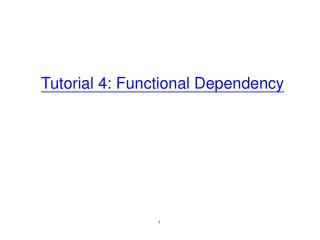DownloadDownload PresentationTutorial 4: Functional Dependency

# Tutorial 4: Functional Dependency

Download Presentation## Tutorial 4: Functional Dependency

- - - - - - - - - - - - - - - - - - - - - - - - - - - E N D - - - - - - - - - - - - - - - - - - - - - - - - - - -
##### Presentation Transcript

1. Tutorial 4: Functional Dependency

2. Question. Let R be a relation with schema {A, B, C, D, E}, on which the following functional dependencies hold: • A  BC, CD  E, B  D, E  A. • Compute the closure of CD. • Also do we have B  E?

3. Let R be a relation with schema {A, B, C, D, E}, on which the following functional dependencies hold: • A  BC, CD  E, B  D, E  A. • Compute the closure of CD. • {CD}+ = {A, B, C, D, E} • Also do we have B  E? • {B}+ = {B, D}. So, no.

4. Question. Let R be a relation with schema {A, B, C, D, E}, on which the following functional dependencies hold: • A  BC, CD  E, B  D, E  A. • List all the candidate keys of R.

5. R = {A, B, C, D, E}. • A  BC, CD  E, B  D, E  A. • Solution sketch: • {A}+= {A, B, C, D, E} • {B}+= {B, D} • {C}+= {C} • {D}+= {D} • {E}+= {A, B, C, D, E} • {A} and {E} are candidate keys. However, there may exist other candidate keys that do not involve these attributes. • {BC}+ = {A, B, C, D, E} • {BD}+ = {B, D} • {CD}+ = {A, B, C, D, E} • {BC} and {CD} are also candidate keys. • Every other combination of attributes fully encloses at least one of the 4 candidate keys already found. So no other candidate key can exist.

6. Question. Consider relation R(title, theater, city) where • title is the name of a movie, • theater is the name of a theater playing the movie, and • city is the city where the theater is located. • We know • Different cities cannot have theaters with the same name. • Different theaters in the same city cannot play the same movie. • Write the functional dependencies implied by the above assumptions. • If we decompose R into (title, theater) and (theater, city), is this a legal decomposition?

7. Different cities cannot have theaters with the same name. • Different theaters in the same city cannot play the same movie. • Functional dependencies: • theatercity • (city, title) theater • If we decompose R into R1(title, theater) and R2(theater, city), is this a legal decomposition? • Yes. • R1 and R2 have a common attribute theater. • This attribute is a candidate key of R2.

8. Question. Consider table TABLE(A, B, C). Write an SQL query to test whether A  B holds. • Given two answers, one containing a nested query and the other not.

9. Consider table TABLE(A, B, C). Write an SQL query to test whether A  B holds. • Answer 2: • SELECT AFROM TABLE T1WHERE EXISTS (SELECT T2.A FROM TABLET2 WHERE T2.A = T1.A and T2.B <> T1.B) • The functional dependency holds if and only if the result is empty.

10. Consider table TABLE(A, B, C). Write an SQL query to test whether A  B holds. • Answer 1: • SELECT AFROM TABLE T1GROUP BY AHAVING COUNT(DISTINCT B) > 1 • The functional dependency holds if and only if the result is empty.